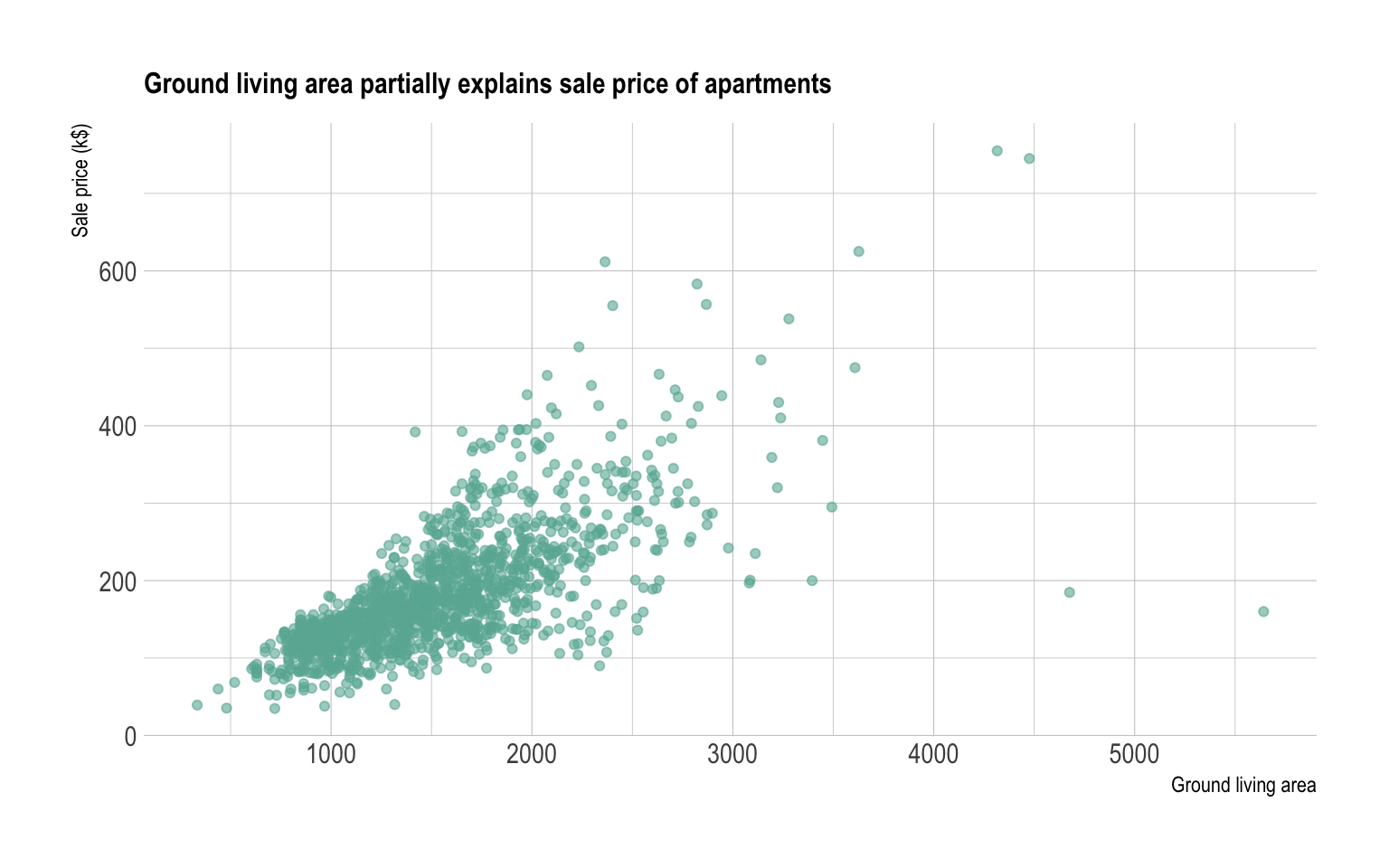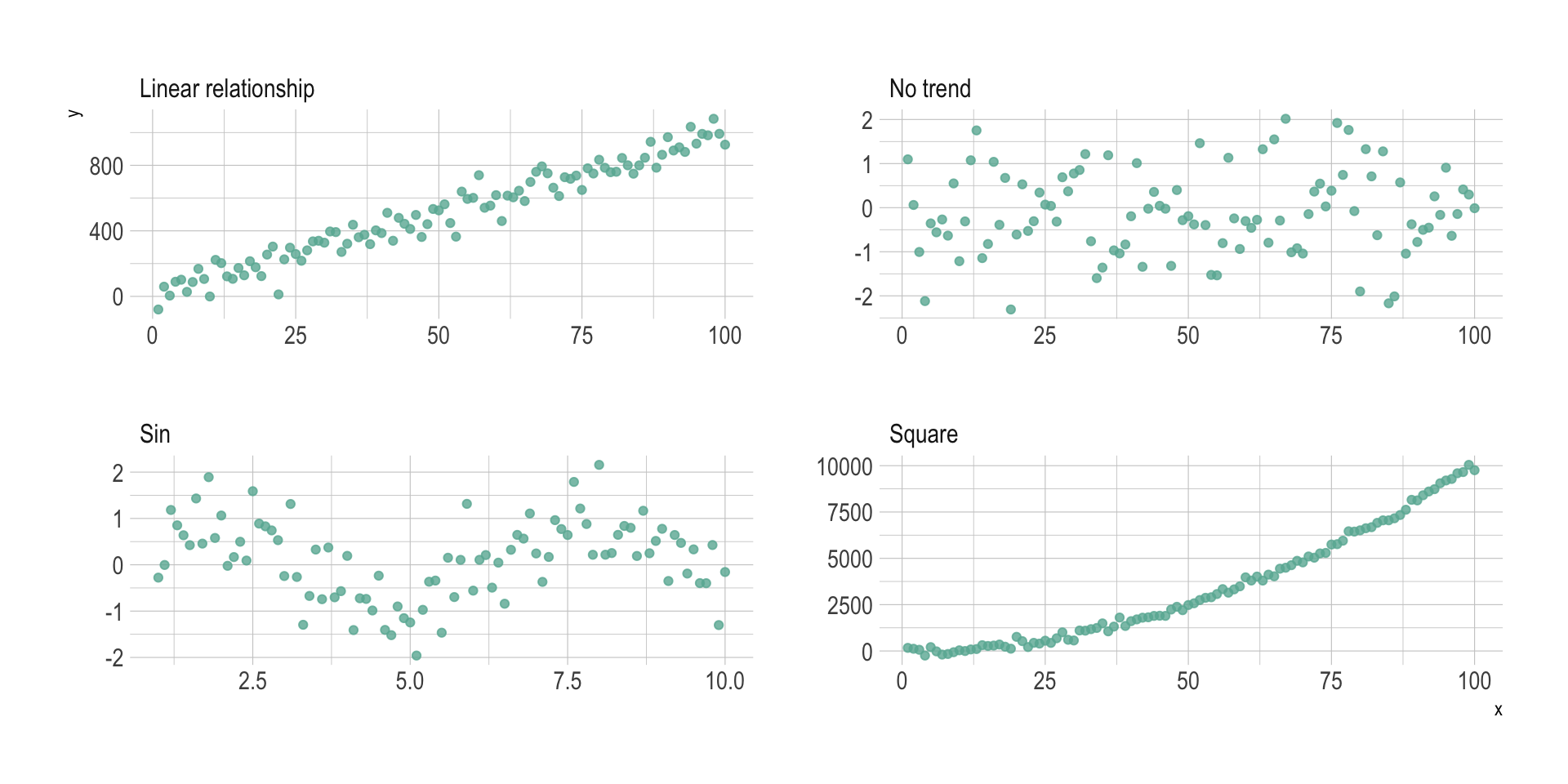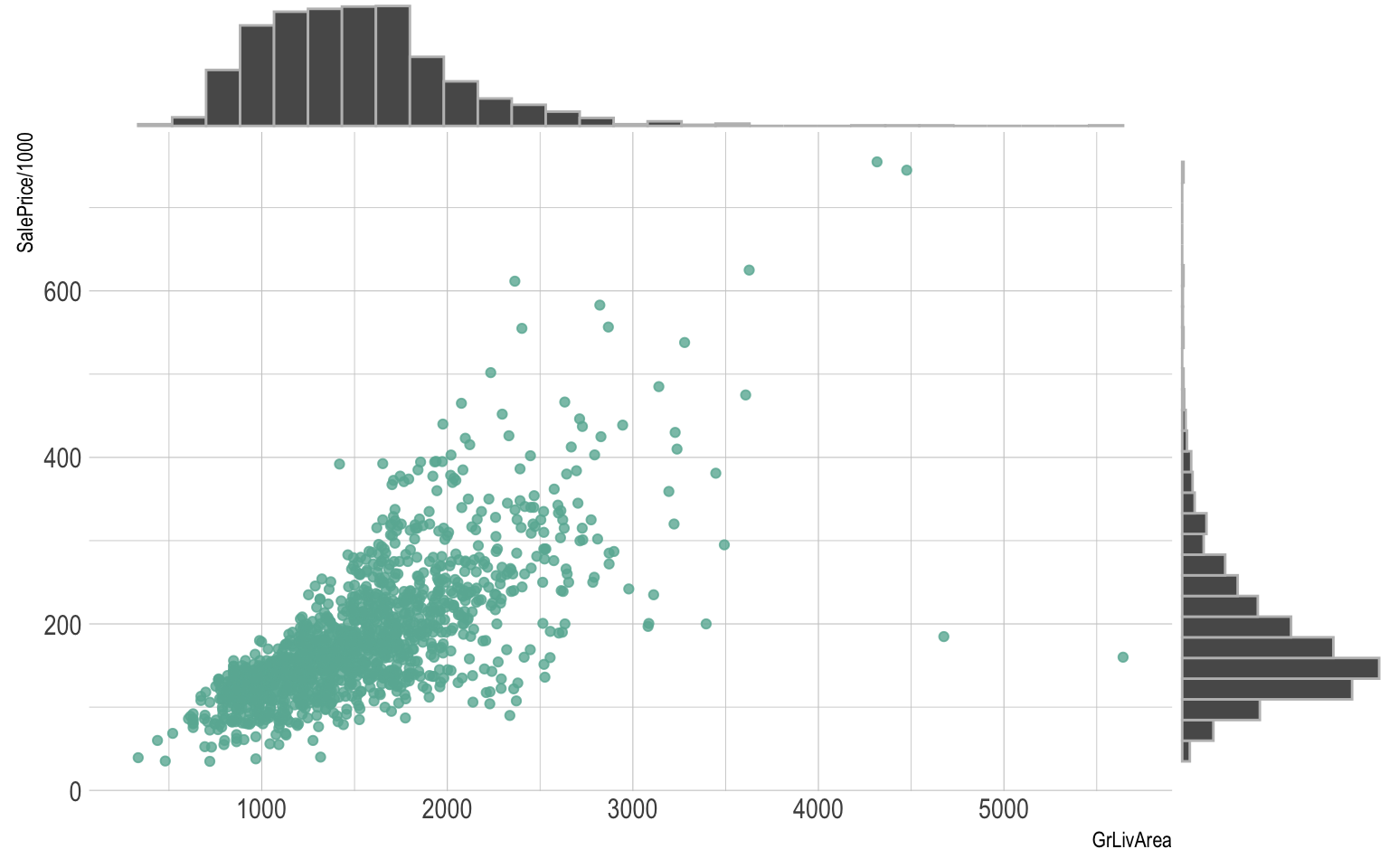# Definition

A scatterplot displays the relationship between 2 numeric variables. For each data point, the value of its first variable is represented on the X axis, the second on the Y axis.

Here is an example considering the price of 1460 apartements and their ground living area. This dataset comes from a kaggle machine learning competition. You can read more about this example here.

``````# Libraries
library(tidyverse)
library(hrbrthemes)
library(viridis)

# Load dataset from github
data <- read.table("https://raw.githubusercontent.com/holtzy/data_to_viz/master/Example_dataset/2_TwoNum.csv", header=T, sep=",") %>% dplyr::select(GrLivArea, SalePrice)

# plot
data %>%
ggplot( aes(x=GrLivArea, y=SalePrice/1000)) +
geom_point(color="#69b3a2", alpha=0.8) +
ggtitle("Ground living area partially explains sale price of apartments") +
theme_ipsum() +
theme(
plot.title = element_text(size=12)
) +
ylab('Sale price (k\$)') +
xlab('Ground living area')``````# What for

A scatterplot is made to study the relationship between 2 variables. Thus it is often accompanied by a correlation coefficient calculation, that usually tries to measure the `linear relationship`.

However other types of relationship can be detected using scatterplots, and a common task consists to fit a model explaining Y in function of X. Here is a few pattern you can detect doing a scatterplot.

``````# Create data
d1 <- data.frame(x=seq(1,100), y=rnorm(100), name="No trend")
d2 <- d1 %>% mutate(y=x*10 + rnorm(100,sd=60)) %>% mutate(name="Linear relationship")
d3 <- d1 %>% mutate(y=x^2 + rnorm(100,sd=140)) %>% mutate(name="Square")
d4 <- data.frame( x=seq(1,10,0.1), y=sin(seq(1,10,0.1)) + rnorm(91,sd=0.6)) %>% mutate(name="Sin")
don <- do.call(rbind, list(d1, d2, d3, d4))

# Plot
don %>%
ggplot(aes(x=x, y=y)) +
geom_point(color="#69b3a2", alpha=0.8) +
theme_ipsum() +
facet_wrap(~name, scale="free")``````# Variation

Interactivity is a real plus for scatterplot. It allows to `zoom` on a specific part of the graphic to detect more precise pattern. It also allows to `hover` dots to get more information about them, like below:

``````# Plotly allows to turn any ggplot2 graphic interactive
library(plotly)

p <- data %>%
mutate(text=paste("Apartment Number: ", seq(1:nrow(data)), "\nLocation: New York\nAny other information you need..", sep="")) %>%
ggplot( aes(x=GrLivArea, y=SalePrice/1000, text=text)) +
geom_point(color="#69b3a2", alpha=0.8) +
ggtitle("Ground living area partially explains sale price of apartments") +
theme_ipsum() +
theme(
plot.title = element_text(size=12)
) +
ylab('Sale price (k\$)') +
xlab('Ground living area')

ggplotly(p, tooltip="text")``````

Scatterplot are sometimes supported by marginal distributions. It indeed adds insight to the graphic, revealing the distribution of both variables:

``````library(ggExtra)

# create a ggplot2 scatterplot
p <- data %>%
ggplot( aes(x=GrLivArea, y=SalePrice/1000)) +
geom_point(color="#69b3a2", alpha=0.8) +
theme_ipsum() +
theme(
legend.position="none"
)

# add marginal histograms
ggExtra::ggMarginal(p, type = "histogram", color="grey")``````# Common mistakes

• Overplotting is the most common mistake when sample size is high. This post describes about 10 different workarounds to fix this issue.

• Don’t forget to show subgroups if you have some. Indeed it can reveal important hidden patterns in your data, like in the case of the Simpson paradox.

# Build your own

The R and Python graph galleries are 2 websites providing hundreds of chart example, always providing the reproducible code. Click the button below to see how to build the chart you need with your favorite programing language.